January 26, 2021, 01:22:27 pm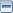Добре дошъл/дошла, Гост. Моля, въведи своето потребителско име или се регистрирай.Изгуби ли регистрационния е-мейл? 1 Час 1 Ден 1 Седмица 1 Месец Завинаги Влез с потребителско име, парола и продължителност на сесията
 Новини: SMF - Just Installed!Заглавна Страница Помощ Търси Календар Вход Регистрирай
 Страници:Автор Тема: Брутален лаг  (Прочетена 12659 пъти) 0 Членове и 1 Гост преглежда(т) тази тема.
alnghost
Jr. Member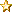Публикации: 56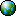« -: November 04, 2007, 09:51:02 pm »

Към всичко, което е чужбина и чат
пат БГ, много лош пинг, загуба на пакети, а на места и интернета
направо спира.Дайте някакъв акъл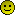.Активен
atcbg
Jr. MemberПубликации: 71« Отговор #1 -: November 04, 2007, 09:52:08 pm »

да и при мен ,вече супер изнервя
вземете
го оправете много време стана
като се спече нета и по 10-15 сек не
може да зареди нищоАктивен
koraba
Jr. MemberПубликации: 60« Отговор #2 -: November 05, 2007, 04:46:01 pm »

има профилактика на интернета и сега са
закупени още 500mbit международен...така че до няколко дни трябва да се
изглади проблемаАктивен

98% от младото поколение е опитвало
трева или някакъв вид опиятия!Ако си от тези 2%, които не са опитвали
сложи това под подписа си!!!
alnghost
Jr. MemberПубликации: 56« Отговор #3 -: November 05, 2007, 05:27:22 pm »

3000 ping, не виждам аз подобрение
засега...Активен
koraba
Jr. MemberПубликации: 60« Отговор #4 -: November 05, 2007, 05:30:35 pm »

обади се в офиса и си кажи юзера и ще бъдат
изпратени технициАктивен

98% от младото поколение е опитвало
трева или някакъв вид опиятия!Ако си от тези 2%, които не са опитвали
сложи това под подписа си!!!
slatina
Администратор
Full Member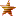Публикации: 222« Отговор #5 -: November 06, 2007, 10:09:32 am »

Извиняваме се на всички засегнати клиенти
на 4.11.2007 около 19.10 h. за наше голямо съжаление един от
централните ни SWICH спря до към 23.45 h. беше подменен ако пак има
проблеми моля звънете на тел. 8710184.
Съжаляваме за причиненото
неудобство.Активен
alnghost
Jr. MemberПубликации: 56« Отговор #6 -: November 07, 2007, 03:41:16 pm »

Не знам какво ви е спряло ама такъв брутален
лаг навсякъде в чужбина е направо достойно за доставчик от ранните зори
на кабелния интернет и нищо не се подобрява вече 10 дни и в офиса
звънях - нищо - отговор тип "уххх, ше ви пратиме техниците"... Техници
не ми трябват до вас съм на 10мс, след вас е проблема
...ЕтоАктивен
slatina
Администратор
Full MemberПубликации: 222« Отговор #7 -: November 07, 2007, 08:35:47 pm »

Нещо не мога да те разбера аз тук не виждам
пробем ....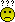До каде ти е БРУТАЛНИЯ ЛАГ??Активен
holymen
NewbieПубликации: 13« Отговор #8 -: January 03, 2008, 01:05:53 am »

слатина-стаф, проблемът явно не е единичен
случай. И в другата тема споделих, и тук ще споделя, че аз го имам този
проблем от 2-3 седмици. И то не само външни сайтове, ами и
български.

Един дир.бг го зарежда по 20-30 секунди, това не е
нормално.

В мое помещение са 2та суича за моят блок, погледнах
ги - всичко свети, всичко уж наред, помислих да не е от мен, но тук
засичам вече, че не съм единственият с проблем.

Дали ще има
някакво подобрение?Активен
stancho
Sr. MemberПубликации: 455« Отговор #9 -: January 03, 2008, 11:34:11 am »

Проблем при мен лично няма с отварянето на
сайтове още повече с Български относно "Бруталния Лаг" аз немога да
разбера кое точно ти лагва. След като ms се качват на 120ms, ако
говориш за това ОК само кажи как да оправим проблем който не е от нас
да отидем и да оправим жиците на целият сват освен друго решение не
виждам. Още повече, че проблема е извън БГ :!: :!: :!: :!:

Без
да обиждам никой, но за мен проблема ви е в задклавиетурното
устройство. Аз сваалям с 1,2-1,5 MB/s външен и 5-6 MB/s BG. сайтовете
се отварят като си цъкна на секундата. Кажете точно какъв е проблема за
да се търси конкретна причина :!: :!: :!: :!:Активен
nobody
phpBB Optic-Com - Staff
NewbieПубликации: 17« Отговор #10 -: January 05, 2008, 04:50:51 pm »

когато имате някакъв проблем със нет-а до
30-40 минути не се оправи зЗззз в офиса, а не после "ми аз нямам нет от
2 седмици" е ние от каде да знаеме, че нямаш като не си се обадил за
проблем?!

Поздрави, HuKouCu :roll:Активен
alnghost
Jr. MemberПубликации: 56« Отговор #11 -: March 19, 2008, 12:52:14 pm »

Сега сигурно, пак си измислям, че ми лаги...
кабела на мегалана ми стига до пред машината имайте го
предвид...

with

Reply from 87.248.113.14: bytes=32 time=86ms TTL=1
from 87.248.113.14: bytes=32 time=93ms TTL=1
Request timed
out.
Request timed out.
Request timed out.
87.248.113.14: bytes=32 time=123ms TTL=1
bytes=32 time=101ms TTL=1
time=95ms TTL=1
TTL=1
Request timed out.
time=99ms TTL=1
TTL=1
Reply from 87.248.113.14: bytes=32 time=105ms TTL=1
from 87.248.113.14: bytes=32 time=116ms TTL=1
87.248.113.14: bytes=32 time=106ms TTL=1

Pinging www.abv.bg
[194.153.145.104] with 32 bytes of d

bytes=32 time=33ms TTL=1
time=28ms TTL=1
TTL=1
Reply from 194.153.145.104: bytes=32 time=24ms TTL=1
from 194.153.145.104: bytes=32 time=32ms TTL=1
194.153.145.104: bytes=32 time=1ms TTL=1
bytes=32 time=3ms TTL=1
Request timed out.
Request timed
out.
Reply from 194.153.145.104: bytes=32 time=7ms TTL=1
from 194.153.145.104: bytes=32 time=27ms TTL=1
194.153.145.104: bytes=32 time=21ms TTL=1
194.153.145.104: bytes=32 time=22ms TTL=1
194.153.145.104: bytes=32 time=27ms TTL=1
194.153.145.104: bytes=32 time=24ms TTL=1Активен
slatina
Администратор
Full MemberПубликации: 222« Отговор #12 -: March 20, 2008, 09:47:18 am »

Е като ти стига кабела до компютъра защо не
пробва през тях да видиш че и там е така........

Първото е пинга
към теб .....Pinging 87.120.239.123 with 1024 bytes of
data:

TTL=1
Reply from 87.120.239.123: bytes=1024 time=4ms TTL=1
from 87.120.239.123: bytes=1024 time=4ms TTL=1
87.120.239.123: bytes=1024 time=4ms TTL=1
bytes=1024 time=4ms TTL=1
time=4ms TTL=1
TTL=1
87.120.239.123: bytes=1024 time=4ms TTL=1
bytes=1024 time=4ms TTL=1
time=4ms TTL=1
TTL=1
Reply from 87.120.239.123: bytes=1024 time=4ms TTL=1
from 87.120.239.123: bytes=1024 time=4ms TTL=1
87.120.239.123: bytes=1024 time=4ms TTL=1
bytes=1024 time=4ms TTL=1
time=6ms TTL=1
TTL=1
Reply from 87.120.239.123: bytes=1024 time=4ms TTL=1
from 87.120.239.123: bytes=1024 time=4ms TTL=1
87.120.239.123: bytes=1024 time=4ms TTL=1
bytes=1024 time=4ms TTL=1
time=4ms TTL=1
TTL=1
Reply from 87.120.239.123: bytes=1024 time=4ms TTL=1
from 87.120.239.123: bytes=1024 time=4ms TTL=1
87.120.239.123: bytes=1024 time=4ms TTL=1
bytes=1024 time=4ms TTL=1
time=4ms TTL=1
TTL=1
Reply from 87.120.239.123: bytes=1024 time=5ms TTL=1
from 87.120.239.123: bytes=1024 time=4ms TTL=1
87.120.239.123: bytes=1024 time=4ms TTL=1
bytes=1024 time=5ms TTL=1
time=4ms TTL=1
TTL=1
Reply from 87.120.239.123: bytes=1024 time=4ms TTL=1
from 87.120.239.123: bytes=1024 time=4ms TTL=1
87.120.239.123: bytes=1024 time=4ms TTL=1
bytes=1024 time=4ms TTL=1
time=4ms TTL=1
TTL=1
Reply from 87.120.239.123: bytes=1024 time=4ms TTL=1
from 87.120.239.123: bytes=1024 time=4ms TTL=1
87.120.239.123: bytes=1024 time=5ms TTL=1
bytes=1024 time=4ms TTL=1
time=4ms TTL=1
TTL=1
Reply from 87.120.239.123: bytes=1024 time=4ms TTL=1
from 87.120.239.123: bytes=1024 time=4ms TTL=1
87.120.239.123: bytes=1024 time=6ms TTL=1
bytes=1024 time=4ms TTL=1
time=4ms TTL=1
TTL=1
Reply from 87.120.239.123: bytes=1024 time=4ms TTL=1
from 87.120.239.123: bytes=1024 time=4ms TTL=1
87.120.239.123: bytes=1024 time=4ms TTL=1
bytes=1024 time=6ms TTL=1
time=4ms TTL=1
TTL=1
Reply from 87.120.239.123: bytes=1024 time=4ms TTL=1
from 87.120.239.123: bytes=1024 time=4ms TTL=1
87.120.239.123: bytes=1024 time=4ms TTL=1
bytes=1024 time=4ms TTL=1
time=4ms TTL=1
TTL=1
Reply from 87.120.239.123: bytes=1024 time=4ms TTL=1
from 87.120.239.123: bytes=1024 time=4ms TTL=1
87.120.239.123: bytes=1024 time=4ms TTL=1
bytes=1024 time=4ms TTL=1
time=4ms TTL=1
TTL=1
Reply from 87.120.239.123: bytes=1024 time=4ms TTL=1
from 87.120.239.123: bytes=1024 time=4ms TTL=1
87.120.239.123: bytes=1024 time=4ms TTL=1
bytes=1024 time=4ms TTL=1

Ping statistics for
87.120.239.123:
Packets: Sent = 75, Received = 75, Lost = 0 (0%
loss),
Approximate round trip times in milli-seconds:
Minimum =
3ms, Maximum = 6ms, Average = 4ms

А второто е пинга към
въпросното место ........

Pinging 87.248.113.14 with 1024 bytes
of data:

TTL=1
Reply from 87.248.113.14: bytes=1024 time=71ms TTL=1
from 87.248.113.14: bytes=1024 time=71ms TTL=1
87.248.113.14: bytes=1024 time=72ms TTL=1
bytes=1024 time=73ms TTL=1
time=72ms TTL=1
TTL=1
Reply from 87.248.113.14: bytes=1024 time=72ms TTL=1
from 87.248.113.14: bytes=1024 time=71ms TTL=1
87.248.113.14: bytes=1024 time=71ms TTL=1
bytes=1024 time=71ms TTL=1
time=72ms TTL=1
TTL=1
Reply from 87.248.113.14: bytes=1024 time=71ms TTL=1
from 87.248.113.14: bytes=1024 time=72ms TTL=1
87.248.113.14: bytes=1024 time=71ms TTL=1
bytes=1024 time=77ms TTL=1
time=74ms TTL=1
TTL=1
Reply from 87.248.113.14: bytes=1024 time=74ms TTL=1
from 87.248.113.14: bytes=1024 time=74ms TTL=1
87.248.113.14: bytes=1024 time=72ms TTL=1
bytes=1024 time=72ms TTL=1
time=71ms TTL=1
TTL=1
Reply from 87.248.113.14: bytes=1024 time=72ms TTL=1
from 87.248.113.14: bytes=1024 time=71ms TTL=1
87.248.113.14: bytes=1024 time=72ms TTL=1
bytes=1024 time=71ms TTL=1
time=72ms TTL=1
TTL=1
Reply from 87.248.113.14: bytes=1024 time=71ms TTL=1
from 87.248.113.14: bytes=1024 time=71ms TTL=1
87.248.113.14: bytes=1024 time=71ms TTL=1
bytes=1024 time=71ms TTL=1
time=72ms TTL=1
TTL=1
Reply from 87.248.113.14: bytes=1024 time=73ms TTL=1
from 87.248.113.14: bytes=1024 time=71ms TTL=1
87.248.113.14: bytes=1024 time=73ms TTL=1
bytes=1024 time=72ms TTL=1
time=71ms TTL=1
TTL=1
Reply from 87.248.113.14: bytes=1024 time=72ms TTL=1
from 87.248.113.14: bytes=1024 time=72ms TTL=1
87.248.113.14: bytes=1024 time=71ms TTL=1

Ping statistics for
87.248.113.14:
Packets: Sent = 46, Received = 46, Lost = 0 (0%
loss),
Approximate round trip times in milli-seconds:
Minimum =
70ms, Maximum = 77ms, Average = 71ms

Сега в момента не
виждам проблем но когато го е имало тогава трябваше да звъънеш ако имаш
рутер виж му настройките и да не се е строшил той нещо ако пак е така
звънни един телефон 02 8710184 и ще долети техник с лаптоп за
установяване на проблема...Активен
 Страници: 
Отиди на:

Страницата е създадена за 0.079 секунди с 18 запитвания.